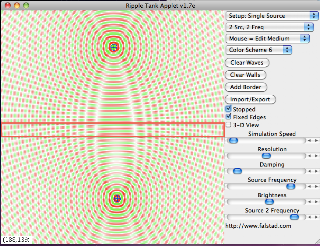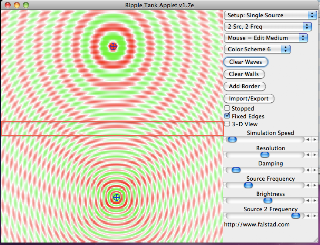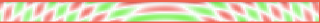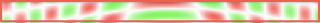Tuesday, February 26, 2013

RTE.2.26 Looking for Gwaves Number 4

If you are interested in the nemetic framework refer to

Nemetical approach to Complex Adaptive Systems – interactions of Red, Green, Blue Waves http://rgbwaves.wordpress.com/ by +Dibyendu DeFigure 1  B Frequency High R Frequency HighFigure 1 BwavesFigure 2  B Frequency = Medium R Frequency = HighFigure 2 BwavesFigure 3 B Frequency  = Low  R Frequency = HighFigure 3 BwavesFigure 4 B Frequency = Low R Frequency = MediumFigure 4 BwavesFigure 5 B Frequency = Low R Frequency = LowFigure 5 Bwaves